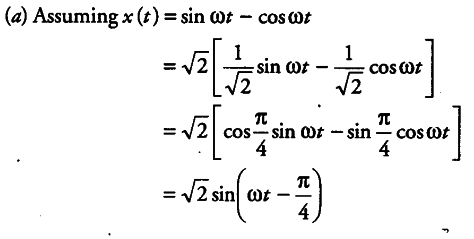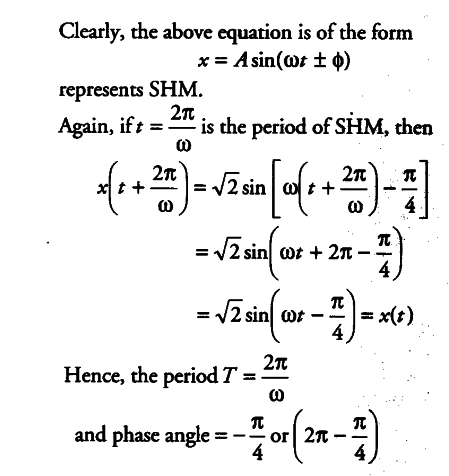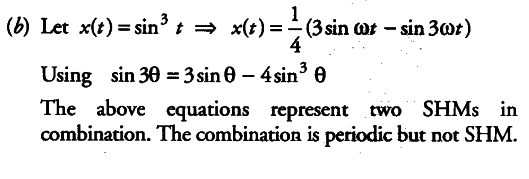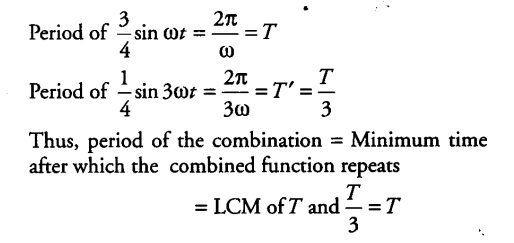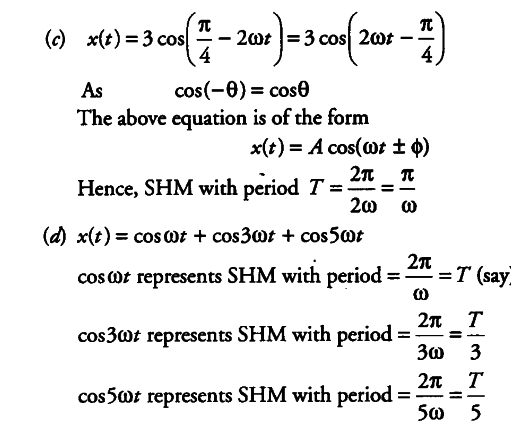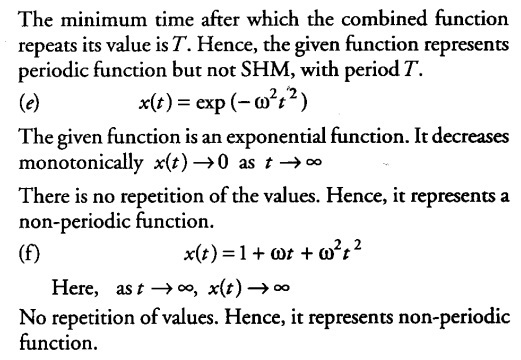# Plot the corresponding reference circle for each of the following

Plot the corresponding reference circle for each of the following simple harmonic motions. Indicate the initial (t = 0) position of the particle, the radius of the circle and the angular speed of the rotating particle. For simplicity, the sense of rotation may be fixed to be anti-clockwise in every case (x is in cm and t is in second).
(a) sinωt -cos ωt
(b)${{sin}^{3}}$ ωt
©3 cos($\pi$ /4 - 2ωt)
(d)cosωt+ 3 cosωt + cos 5ωt
(e) exp(${{-ω}^{2}}$ ${{t}^{2}}$
(f)1+ ωt + ${{ω}^{2}}$ ${{t}^{2}}$